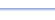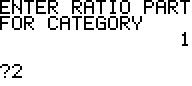Program: RATIO

RATIO

• Finds the number of items in each group, given the ratio and total number of items.

Useful for:

• SAT
• ACT
• Algebra 1
• Geometry

See full list of programs

Try these problems using RATIO:
on MathPro Q&A Forum
in SAT and ACT Prep booksExample

Q: A bag contains only red, blue, and green marbles.  The bag contains 40 marbles, and the ratio of red marbles to blue marbles to green marbles is 2:3:5.  How many green marbles are in the bag?

Solution:  Run RATIO.  How?
Enter 40 for the total number.
Enter 3 for the number of
categories (red, blue, green).

Now, enter the ratio parts
(2, 3, and 5) in order:

The program reports
the distribution of the
40 marbles into the
ratio 2:3:5.

Press 0 and ENTER to
end the program.

Interpretation:  'Green'
is the third part, so there
are 20 green marbles.

Note:  When the list is long, the
numbers may scroll off the right
side of the screen.  Choose 1 in
the last screen, and press ENTER
repeatedly to list the numbers one at a time.